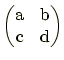### General single-qubit unitary operation

This command is used to apply a given 1-qubit operation to the qubits specified by bit labels. Format:
U (bit labels, (a b)(c d));
where bit labels are given by the text in the style of 1-6'' (qubit 1 to qubit 6) and/or 1 3 5'' (qubit 1, 3, and 5), and (a b)(c d) is regarded as the matrix. a,...,d may be complex numbers. Example:
U (1 2 4-7, (0 -i)(i 0));
When a user defines a matrix A by matrix A (a b)(c d);'' (See also Sec. 7.1) prior to the invocation of U(...), then
U(bit labels, A);
may be used. However, in this case, one should use
A(bit labels);
The matrix may be an intrinsic matrix X'', Rx(angle)'', etc., e.g.,
U(1-3, Rx(45deg));

root
2004-06-15